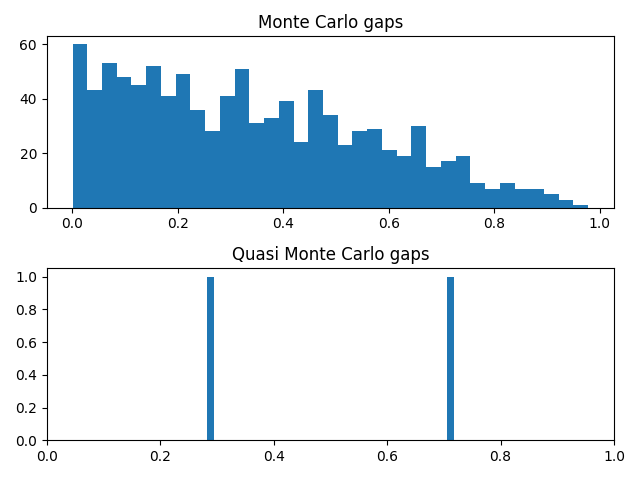# The quality of an RNG depends on the application

A random number generator can be good for some purposes and not for others. This isn’t surprising given the fundamentally impossible task such generators are supposed to perform. Technically a random number generator is a pseudo random number generator because it cannot produce random numbers. But random is as random does, and for many purposes the output of a pseudorandom number generator is random for practical purposes. But this brings us back to purposes.

Let β be an irrational number and consider the sequence

xnnβ mod 1

for n = 0, 1, 2, … That is, we start at 0 and repeatedly add β, taking the fractional part each time. This gives us a sequence of points in the unit interval. If you think of bending the interval into a circle by joining the 1 end to 0, then our sequence goes around the circle, each time moving β/360°.

Is this a good random number generator? For some purposes yes. For others no. We’ll give an example of each.

## Integration

If your purpose is Monte Carlo integration, then yes it is. Our sequence has low discrepancy. You can approximate the integral of a function f over [0, 1] by taking the average of f(xn) over the first N elements of the sequence. Doing Monte Carlo integration with this particular RNG amounts to quasi Monte Carlo (QMC) integration, which is often more efficient than Monte Carlo integration.

Here’s an example using β = e.

```    import numpy as np

# Integrate f with N steps of (quasi) Monte Carlo
def f(x): return 1 + np.sin(2*np.pi*x)
N = 1000

# Quasi Monte Carlo
sum = 0
x = 0
e = np.exp(1)
for n in range(N):
sum += f(x)
x = (x + e) % 1
print(sum/N)

# Monte Carlo
sum = 0
np.random.seed(20220623)
for _ in range(N):
sum += f(np.random.random())
print(sum/N)
```

This code prints

```    0.99901...
0.99568...
```

The exact value of the integral is 1, and so the error using QMC between 4 and 5 times smaller than the error using MC. To put it another way, integration using our simple RNG is much more accurate than using the generally better RNG that NumPy uses.

## Simulation

Now suppose we’re doing some sort of simulation that requires computing the gaps between consecutive random numbers. Let’s look at the set of gaps we get using our simple RNG.

```    gapset = set()
x = 0
for _ in range(N):
newx = (x + e) % 1
gap = np.abs(newx - x)
x = newx
print( gapset )
```

Here we rounded the gaps to 10 decimal places so we don’t have minuscule gaps caused by floating point error.

And here’s our output:

`    {0.7182818285, 0.2817181715}`

There are only two gap sizes! This is a consequence of the three-gap theorem that Greg Egan mentioned on Twitter this morning. Our situation is a slightly different in that we’re looking at gaps between consecutive terms, not the gaps that the interval is divided into. That’s why we have two gaps rather than three.

If we use NumPy’s random number generator, we get 1000 different gap sizes.## Cryptography

Random number generators with excellent statistical properties may be completely unsuitable for use in cryptography. A lot of people don’t know this or don’t believe it. I’ve seen examples of insecure encryption systems that use random number generators with good statistical properties but bad cryptographic properties.

These systems violate Kirchoff’s principle that the security of an encryption system should reside in the key, not in the algorithm. Kirchoff assumed that encryption algorithms will eventually be known, and difficult to change, and so the strength of the system should rely on the keys it uses. At best these algorithms provide security by obscurity: they’re easily breakable knowing the algorithm, but the algorithm is obscure. But these systems may not even provide security by obscurity because it may be possible to infer the algorithm from the output.

## Fit for purpose

The random number generator in this post would be terrible for encryption because the sequence is trivially predictable. It would also fail some statistical tests, though it would pass others. It passes at least one statistical test, namely using the sequence for accurate Monte Carlo integration.

Even so, the sequence would pass a one-sided test but not a two-sided test. If you tested whether the sequence, when used in Monte Carlo integration, produced results with error below some threshold, it would pass. But if you looked at the distribution of the integration errors, you’d see that they’re smaller than would be expected from a random sequence. The sequence provides suspiciously good integration results, failing a test of randomness but suggesting the sequence might be useful in numerical integration.

## One thought on “The quality of an RNG depends on the application”

1. Stan Alekman

Your post reminded me of something humorous I read years ago, “Random numbers are too important to be left to chance alone.”

Unfortunately I do not remember the source.